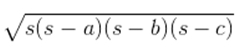Guru

# An isosceles triangle has perimeter 30 cm and each of the equal sides is 12 cm. Find the area of the triangle. Q.6

• 0

Please give me the best way for solving the problem of class 9th ncert math of Heron’s Formula chapter of math of class 9th of exercise 12.1 of question no 6 what is the tricky way for solving this question An isosceles triangle has perimeter 30 cm and each of the equal sides is 12 cm. Find the area of the triangle.

Share

1. First, let the third side be x.

It is given that the length of the equal sides is 12 cm and its perimeter is 30 cm.

So, 30 = 12+12+x

∴ The length of the third side = 6 cm

Thus, the semi perimeter of the isosceles triangle (s) = 30/2 cm = 15 cm

Using Heron’s formula,

Area of the triangle

== √[15(15-12)(15-12)(15-6)] cm2

= √[15×3×3×9] cm2

= 9√15 cm2

• 0